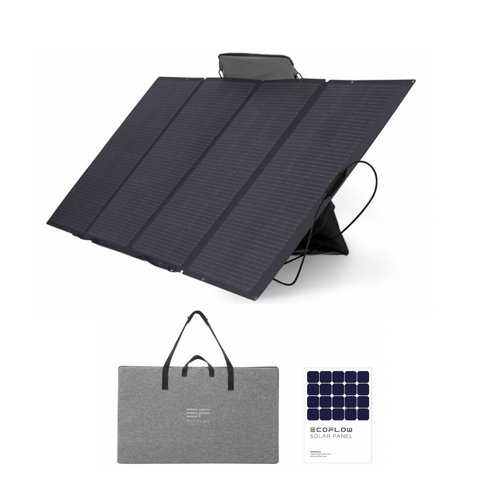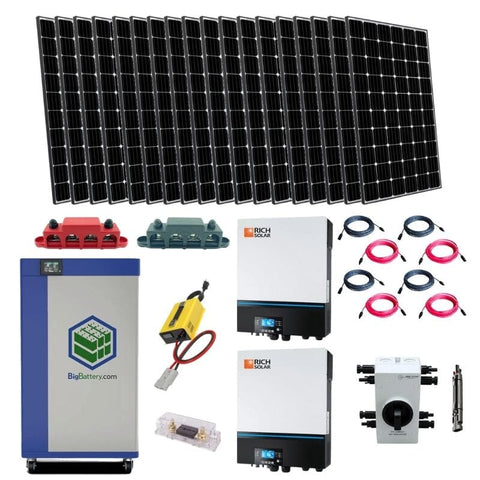Solar Panel Square Footage Calculator (Guide for Solar Sizing) - ShopSolar.com

# Solar Panel Square Footage Calculator

Calculating the exact square footage needed for your solar panels is the first step you need to take before heading out and purchasing a rooftop solar power system.

To determine the total square footage required, simply take the # of solar panels you have and multiply it by 17.55 square feet. This is the average size of residential solar panels and will give you a very close estimate of the total square footage you need for your solar panels.

For example, if we needed 27 solar panels for our system:

Square Footage =  27*17.55 = 473.85 square feet

Most first-time buyers make the mistake of not calculating the number of solar panels needed, which has the potential of causing a few problems during installation.

While there isn’t a lot wrong with initially focusing on square footage, the process of installation becomes a lot easier once you have an idea of the number of panels needed to power your home independently.

There is a lot of complexity involved in square footage calculations and unfortunately, there isn’t one simple equation that can quickly determine how much space is needed for a certain number of panels.

Having a good understanding of the intricacies involved in square footage calculations and solar panel roof size calculators will make your installation process stress free.

Every home is different from the next which means that solar requirements will change depending on the homeowner's needs.

In this article you will learn everything there is to know about solar panel square footage, as well as things to consider for a successful solar panel system.

## Solar Panel Wattage CalculatorBefore we can determine the number of panels needed in line with our square footage, we first need to know the wattage of our solar panels.

We’re going to need this metric later to calculate the roof space needed for a successful installation.

Thankfully, you don’t need to use a solar panel watt calculator to determine the wattage rating of your solar panel, as all newly purchased panels will have the rating somewhere on the packing or manual.

If you have a hard time locating it, then try to look for the power rating, which is another term given to solar wattage.

To save time, most modern panels have a wattage or power rating of 370 watts. It is a good rule of thumb to use this reading for solar calculations, especially when working out the number of panels you are going to need for a specific system size.

To make our calculations easy to understand, we will be using the 370-watt rating.

### 10 kW Solar System Calculator

Square footage calculations can be confusing and overcomplicated with a ton of unnecessary math. So, before we get into that we thought we should ease you in by showing you how to calculate how many solar panels are needed for a specific-sized panel system.

10kW systems are the go-to for many residential homes and they will ensure that you are provided with a sufficient amount of electricity throughout the day.

Suppose you are aiming for a 10kW system without having calculated the actual size needed. In that case, we are going to show you how to easily calculate the number of panels needed to ensure that your 10kW system is running efficiently.

Divide the size of the solar system by the wattage of each panel to calculate the number of panels required. Remember that we are using 370 watts, so you will use this number to determine the number of panels.

To achieve a 10kW solar system you are going to take 10,000 watts (10kW) and divide it by the wattage of a single solar panel (370 watts).

This will give you a reading of 27.02, which we round down to 27. Therefore, we need 27 panels for a 10kW solar panel system.

## How Many Solar Panels Do I Need to Power My House?If you don’t have a set solar system size in mind but rather want to work out the number of panels needed to power your home, then you will need to follow a few simple steps.

### Annual Energy Usage

The very first thing you need to do is determine your annual energy usage in kilowatts. Your annual energy usage is dependent on your home appliances that run on electricity and how often they are used.

There are several ways you can calculate your annual energy usage, but the easiest and fastest way would be to find a monthly utility and multiply it by 12 to get your yearly usage.

This gives you a rough estimate, so if you want a more accurate reading, I suggest holding onto your utility bill for each month and adding it up once you have all 12.

If you are that person that throws slips away, don’t worry as you can use the average energy usage for the household which is around 11,000kWh per year. If you want to be more accurate you can use 10,649 kWh per year.

### Solar Peak HoursOnce you have your annual energy down to the tee, you need to find out how much energy a solar panel will produce in your area or location.

To do this you can look up the average sun peak hours in your state and multiply it by 30 to get the monthly reading.

Households in sunny states receive between 130 and 150 peak sun hours a year. Your solar peak hours correlate directly to the amount of electricity that can be produced.

For example, if your panels receive 120 monthly peak sunlight hours, then 1kW of solar will produce 120 kWh of electricity per month.

### Calculate the Solar System Size

Now that we have the energy readings and we roughly know how much energy can be produced, we need to work out the size of our entire solar system.

To do this you will need monthly electricity, which we would have already had from working out our yearly usage. You will then divide your monthly electricity usage by the peak sun hours in your area.

For example, let's say we consumed 900kWh of energy for one whole year and our peak sun house is 120. Simply take the 900kWh and divide it by the 120. This gives us a reading of 7.5kWh of solar.

Therefore, we would need a 7.5kW solar system to meet our energy requirements.

### Determine the Number of PanelsWe now have everything we need to determine the number of panels required for our system. Firstly, you will have to convert the 7.5 kW reading to watts, which you can do by simply multiplying it by 1000.

This would give us 7,500 kW of solar power.

Next, you would then take this number and divide it by the wattage of the panels you are going to have installed. Here we will use the 370 watts we used previously for a single panel.

Doing this calculation will give you a reading of 20.27, which we round down to 20 solar panels.

That’s it! You now have the number of panels needed to power your home.

#### Alternative Method

This is a quicker method of calculating how many solar panels are needed. You will still use your yearly energy usage along with the wattage of a single panel. However, in addition, you will now incorporate the production ratio of your solar panel.

There is a general estimate for production ratios which is usually given as 1.3 or 1.6. You can also work out your production ratios by taking your wattage and dividing it by the electricity output for one year.Then you would use this simple equation to determine the number of panels you need:

Number of Panels Needed = kWh usage/production ratio/wattage

For example, let’s say a household consumes 13,000 kWh of energy in a year. We can then use the estimated production ratio of 1.3 and a panel wattage of 370.

Plugging it all into the formula will give us a panel number of 27.02, which we round down to 27 solar panels.

## How Much Square Foot Do I Need

Now that you have the number of panels you need, the final part of it all is to figure out how much space you need for them to be installed successfully.

On average, it is recommended that you have between 290 and 360 square feet available on your roof for solar panel installation.

To determine the required roof space, simply take the number of panels you need and multiply it by 17.55 square feet. This is the average size of residential panels and will give you a rough estimate of the space you need.

So, for the example we used above, we needed 27 solar panels for our system. To work out the space simply take the 27 and multiply it by 17.55 square feet. This will give us a reading of 473.85 square feet.

It is also beneficial to try a solar panel roof load calculator to determine the stress that your roof can take before installation.

### How to Reduce Amount of Panels Needed

Of course, not all homes have the same amount of space. Some homes are smaller than others and therefore have little roof space to work with when wanting to install solar panels.

Not to worry, there is a solution that can solve the lack of space situation. Firstly, you can purchase panels that have a stronger wattage reading.This means that you will need fewer panels to achieve that same energy output. The EcoFlow 400W monocrystalline panel is a great alternative to your usual 350-watt residential panels. It is a lot more powerful so you will need a lot less to achieve your energy needs.

Another solution would be to consider having a ground-mounted panel system. Ground-mounted solar panels can be installed on any open space on your property with direct sun exposure. It is a great alternative if you don’t have bucketloads of space on your roof.

Lastly, you can turn toward enrolling in a community solar garden or an off-grid solar power system. This is a great solution for those who don’t have lots of roof space and have large areas of shading. Solar gardens provide solar energy to subscribers and are installed on an open plot of land.## Conclusion

Figuring out the required solar panel square footage can be a daunting task, especially for first-time buyers entering the solar space. However, with our guide, you would experience a lot more success than headaches.

The process can be lengthy, but it is well worth it in the end and will ensure that your solar system can be installed successfully without damage.

After reading this tutorial and if you require a little bit of additional guidance, then you should contact Shop Solar Kits. Along with providing 5-star customer service, we have an amazing selection of solar items, including the potent EP500 Bluetti.And just like that, you are now a master of calculating the square footage required for solar panel installation. You now have the expertise to broaden your knowledge and try out the solar panel amps calculator or if you want to know about cost try the solar panel cost calculator.

Did You Find Our Blog Helpful? Then Consider Checking: